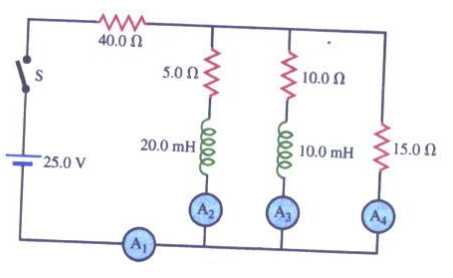# Problem: In the circuit shown in the figure below, switch S is closed at a time t = 0.Find the reading of each ammeter just after the switch is closed.

###### FREE Expert Solution

Ohm's law:

$\overline{){\mathbf{V}}{\mathbf{=}}{\mathbf{i}}{\mathbf{R}}}$

Equivalent resistance for resistors in series:

$\overline{){{\mathbf{R}}}_{{\mathbf{eq}}}{\mathbf{=}}{{\mathbf{R}}}_{{\mathbf{1}}}{\mathbf{+}}{{\mathbf{R}}}_{{\mathbf{2}}}{\mathbf{+}}{\mathbf{.}}{\mathbf{.}}{\mathbf{.}}{\mathbf{+}}{{\mathbf{R}}}_{{\mathbf{n}}}}$

An inductor has infinite resistance at t = 0s. Therefore, it acts as an open circuit.

82% (158 ratings)###### Problem DetailsIn the circuit shown in the figure below, switch S is closed at a time t = 0.

Find the reading of each ammeter just after the switch is closed.

Frequently Asked Questions

What scientific concept do you need to know in order to solve this problem?

Our tutors have indicated that to solve this problem you will need to apply the LR Circuits concept. You can view video lessons to learn LR Circuits. Or if you need more LR Circuits practice, you can also practice LR Circuits practice problems.

What professor is this problem relevant for?

Based on our data, we think this problem is relevant for Professor Murray's class at GT.